## Line 4 Build Codes.

Build codes run from line 3, 4 and 5. Most of the numbers are the last few digits of the factory production part numbers.

VAC FIT: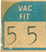The last two digits of the part number for the power brake vacuum fitting.

01 = 3580201 3-way w/ thermostatic ingnition
02 = 2580202 4-way w/ thermostatic ingnition
04 = 3461304 2-way
11 = 3461304 3-way
23 = 3461323 3-way w/o AC
38 = 1948838 3-way w/ AC
54 = 1944154 1-way
66 = 2405466 2-way .247 tube
92 = 1944092 2-way .122 tube

ENGINE: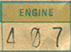The last three digits of the assembly line part number.

382 = 340 4BBL, manual trans., heater, 275hp
383 = 340 4BBL, manual trans., A/C, 275hp
407 = 426 Hemi, manual trans., heater, 425hp
408 = 426 Hemi, auto. trans., heater, 425hp
412 = 440 6BBL, manual trans., heater, 385hp
413 = 440 6BBL, auto. trans., heater, 385hp
418 = 383 4BBL, manual trans., heater, 300hp
419 = 383 4BBL, auto. trans., heater, 300hp
420 = 383 4BBL, auto. trans., A/C, 300hp
443 = 440 4BBL, manual trans., heater, 370hp
444 = 440 4BBL, auto. trans., heater, 370hp
445 = 440 4BBL, auto. trans., A/C, 370hp
451 = 340 4BBL, auto. trans., heater, 275hp
452 = 340 4BBL, auto. trans., A/C, 275hp

CARB: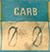The last two digits of the assembly line part number, not the part number used in catalogs. This number will be used with reference of the engine, transmission, with or with out freash air hood, and with or with out AC.

30 = 383 4bbl Auto w/AC
32 = 440 4bbl 4spd
34 = 440 6pk 4spd w/Ramcharger
35 = 440 6pk Auto w/Ramcharger
42 = 383 4bbl Auto w/Ramcharger
48 = 383 4bbl 4spd
50 = 383 4bbl Auto w/Ramcharger
00 = 426 2 4bbl Auto w/Ramcharger

TRANS: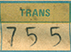The last three digits of the assembly line and catalog part number for auto transmissions.

804 = 3515804 225 CI
806 = 3515806 318 CI
817 = 3515817 440 CI
841 = 3515841 225 CI HD
842 = 3515842 318 CI HD
843 = 3515843 340 CI
845 = 3515845 383 CI 2bbl
846 = 3515846 383 CI 4bbl
847 = 3515847 440 CI Std
848 = 3515848 440 CI HP
849 = 3515849 426 CI
850 = 3515850 440 CI 6pk
851 = 3515851 340 CI E-body
852 = 3515852 318 CI

PIN: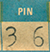The Speedo pinion gear code last two digits.

24 = 3410024
25 = 3410025
26 = 2538926
27 = 2538927
28 = 2538928
29 = 2538929
30 = 2538930
31 = 2538931
32 = 2538932
33 = 2538933
34 = 2538934
35 = 2538935
36 = 2538936
37 = 2538937
38 = 2538938
39 = 2538939
40 = 2538940
41 = 2538941
42 = 2538942
43 = 2538943

P/SHAFT: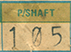The Popeller Shaft, last three digits of part number.

101 = 2996101 50.68 x 3.25
105 = 2996109 49.50 x 3.25
109 = 2996109 50.80 x 3.25
113 = 2996113 53.30 x 3.25
117 = 2996117 53.24 x 3.25
121 = 2996121 57.42 x 3.00
130 = 2991130 50.80 x 3.00
186 = 2996186 53.30 x 3.00
201 = 2996201 54.92 x 3.00
207 = 2996207 54.92 x 3.25
211 = 2996211 57.10 x 3.00
216 = 2996219 57.10 x 3.00
228 = 2996228 59.60 x 3.00
696 = 2883696 53.18 x 3.25
735 = 2883735 57.42 x 3.00

AXLE: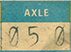The axle code, last three digits of part number. This number is also found on the Lynch Rd Fender tag.

042 = 3.23 open, 8 3/4, 10" rear drum - front disc/LD drum
043 = 3.23 S/G, 8 3/4, 10" rear drum - front disc/LD drum
044 = 3.23 open, 8 3/4, 11" rear & front drums
045 = 3.23 S/G, 8 3/4, 11" rear & front drums
046 = 3.55 S/G, 8 3/4, 11" rear & front drums, (A36 Pkg)
047 = 3.55 S/G, 8 3/4, 10" rear drum - front disc, (A36 Pkg)
048 = 3.91 S/G, 8 3/4, 11" rear & front drums, (A31 Pkg)
049 = 3.91 S/G, 8 3/4, 10" rear drum - front disc, (A31 Pkg)
050 = 3.45 S/G, DANA 60, 11" rear & front drums, (A33 Pkg)
051 = 3.54 S/G, DANA 60, 10" rear drum - front disc, (A33 Pkg)
052 = 4.10 S/G, DANA 60, 10" rear drum - front disc, (A34 Pkg)

WHEELS: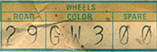Wheels used three boxes grouped under the heading WHEELS.

The First box is for the type of wheel used on the vehicle.

The Second box is the color of the wheel.
NOTE: If full wheel covers are used, the the wheels would be black. If Dog Dish covers are used, in most cases the wheels would be body color. Most Spare wheels are also black unless special spare was ordered or again body color if standard hubcaps were used.

The Third box is used for the type of SPARE wheel used.

ROAD: The last two digits of part number

50 = 2944450 15x7 Steel Wheel
74 = 2944474 14x5.5 Road Wheel
90 = 2944390 15x7 Rally Wheel
78 = 3420978 14x6 Steel Wheel

COLOR:

TX9 = Black
GW3 = White

SPARE: The last two digits of part number

50 = 2944450 15x7 Steel Wheel
78 = 3420978 14x6 Steel Wheel

WHEEL COVER: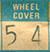The last two digits of part number.

54 = 2944454 Standard hub cap aluminum
22 = 3461222 15" Trim ring
52 = 3461352 Rally center cap

FRAME: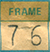The last two digits of K-frame part number.

FT S/BAR: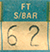The last two digits of Sway Bar part number.

62 = 2835862 .88 Dia. B-body

SHOCK FT (Front) RR (Rear):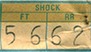The last two digits of factory part number.

56 = 3400556 Front B-Body HD
62 = 3402262 Rear B-Body HD

1: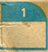UNKNOWN at this time for 1971.